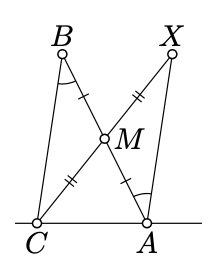$$\newcommand{\id}{\mathrm{id}}$$ $$\newcommand{\Span}{\mathrm{span}}$$ $$\newcommand{\kernel}{\mathrm{null}\,}$$ $$\newcommand{\range}{\mathrm{range}\,}$$ $$\newcommand{\RealPart}{\mathrm{Re}}$$ $$\newcommand{\ImaginaryPart}{\mathrm{Im}}$$ $$\newcommand{\Argument}{\mathrm{Arg}}$$ $$\newcommand{\norm}{\| #1 \|}$$ $$\newcommand{\inner}{\langle #1, #2 \rangle}$$ $$\newcommand{\Span}{\mathrm{span}}$$

# 7.4: Angles of triangles

$$\newcommand{\vecs}{\overset { \rightharpoonup} {\mathbf{#1}} }$$ $$\newcommand{\vecd}{\overset{-\!-\!\rightharpoonup}{\vphantom{a}\smash {#1}}}$$$$\newcommand{\id}{\mathrm{id}}$$ $$\newcommand{\Span}{\mathrm{span}}$$ $$\newcommand{\kernel}{\mathrm{null}\,}$$ $$\newcommand{\range}{\mathrm{range}\,}$$ $$\newcommand{\RealPart}{\mathrm{Re}}$$ $$\newcommand{\ImaginaryPart}{\mathrm{Im}}$$ $$\newcommand{\Argument}{\mathrm{Arg}}$$ $$\newcommand{\norm}{\| #1 \|}$$ $$\newcommand{\inner}{\langle #1, #2 \rangle}$$ $$\newcommand{\Span}{\mathrm{span}}$$ $$\newcommand{\id}{\mathrm{id}}$$ $$\newcommand{\Span}{\mathrm{span}}$$ $$\newcommand{\kernel}{\mathrm{null}\,}$$ $$\newcommand{\range}{\mathrm{range}\,}$$ $$\newcommand{\RealPart}{\mathrm{Re}}$$ $$\newcommand{\ImaginaryPart}{\mathrm{Im}}$$ $$\newcommand{\Argument}{\mathrm{Arg}}$$ $$\newcommand{\norm}{\| #1 \|}$$ $$\newcommand{\inner}{\langle #1, #2 \rangle}$$ $$\newcommand{\Span}{\mathrm{span}}$$

Theorem $$\PageIndex{1}$$

In any $$\triangle ABC$$, we have

$$\measuredangle ABC + \measuredangle BCA + \measuredangle CAB \equiv \pi.$$

Proof

First note that if $$\triangle ABC$$ is degenerate, then the equality follows from Corollary 2.4.1. Further we assume that $$\triangle ABC$$ is nondegenerate.Let $$X$$ be the reflection of $$C$$ across the the midpoint $$M$$ of $$[AB]$$. By Proposition 7.2.1 $$\measuredangle BAX = \measuredangle ABC$$. Note that $$(AX)$$ is a reflection of $$(CB)$$ across $$M$$; therefore by Theorem 7.2.1, $$(AX) \parallel (CB)$$.

Since $$[BM]$$ and $$[MX]$$ do not intersect $$(CA)$$, the points $$B, M$$, and $$X$$ lie on the same side of $$(CA)$$. Applying the transversal property for the transversal $$(CA)$$ to $$(AX)$$ and $$(CB)$$, we get that

$\measuredangle BCA + \measuredangle CAX \equiv \pi.$

Since $$\measuredangle BAX = \measuredangle ABC$$, we have

$$\measuredangle CAX \equiv \measuredangle CAB + \measuredangle ABC$$

The latter identity and 7.4.1 imply the theorem.

Exercise $$\PageIndex{1}$$Let $$\triangle ABC$$ be a nondegenerate triangle. Assume there is a point $$D \in [BC]$$ such that

$$\measuredangle BAD \equiv \measuredangle DAC, BA = AD = DC.$$

Find the angles of $$\triangle ABC$$.

Hint

Apply twice Theorem 4.3.1 and twice Theorem $$\PageIndex{1}$$.

Exercise $$\PageIndex{2}$$

Show that

$$|\measuredangle ABC| + |\measuredangle BCA| + |\measuredangle CAB| = \pi$$

for any $$\triangle ABC$$.

Hint

If $$\triangle ABC$$ is degenerate, then one of the angle measures is $$\pi$$ and the other two are 0. Hence the result.

Assume $$\triangle ABC$$ is nondegenerate. Set $$\alpha = \measuredangle CAB$$, $$\beta = \measuredangle ABC$$, and $$\gamma = \measuredangle BCA$$.

By Theorem 3.3.1, we may assume that $$0 < \alpha, \beta, \gamma < \pi$$. Therefore,

$0 > \alpha + \beta + \gamma < 3 \cdot \pi.$

By Theorem $$\Pageindex{1}$$,

$\alpha + \beta +\gamma \equiv \pi.$

From 7.4.2 and 7.4.3 the result follows.

Exercise $$\PageIndex{3}$$

Let $$\triangle ABC$$ be an isoseles nondegenerate triangle with the base $$[AC]$$. Suppose $$D$$ is a reflection of $$A$$ across $$B$$. Show that $$\angle ACD$$ is right.Hint

Apply twice Theorem 4.3.1 and twice Theorem $$\PageIndex{1}$$.

Exercise $$\PageIndex{4}$$

Let $$\triangle ABC$$ be an isosceles nondegenerate triangel with base $$[AC]$$. Assume that a circle is passing thru $$A$$, centered at a point on $$[AB]$$, and tangent to $$(BC)$$ at the point $$X$$. Show that $$\measuredangle CAX = \pm \dfrac{\pi}{4}$$.

HintSuppose that $$O$$ denotes the center of the circle.

Note that $$\triangle AOX$$ is isosceles and $$\angle OXC$$ is right. Applying Theorem $$\PageIndex{1}$$ and Theorem 4.3.1 and simplifying, we should get $$4 \cdot \measuredangle CAX \equiv \pi$$.

Show that $$\angle CAX$$ has to be acute. It follows then that $$\measuredangle CAX = \pm \dfrac{\pi}{4}$$.

Exercise $$\PageIndex{5}$$

Show that for any quadrangle $$ABCD$$, we have

$$\measuredangle ABC + \measuredangle BCD + \measuredangle CDA + \measuredangle DAB \equiv 0$$.

Hint

Apply Theorem $$\PageIndex{1}$$ to $$\triangle ABC$$ and $$\triangle BDA$$.